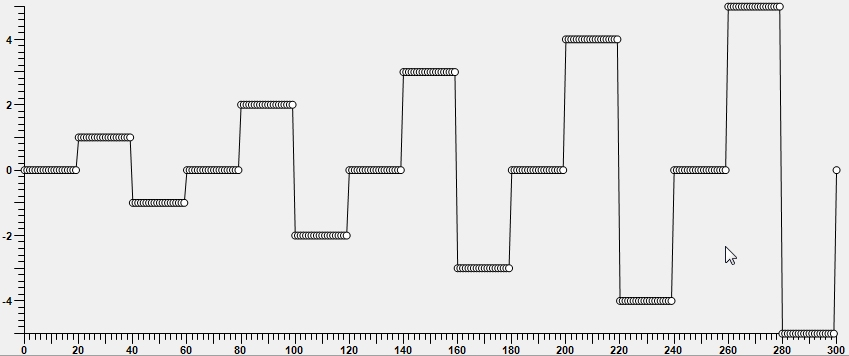Omega software: Waveforms

Measurements may require user specified waveforms, and the expression parser in Omega software is powerful enough to create almost any type of wave forms. This is quick reference index to some typical wave forms, from index 0 to 100.

Creating your own wave forms is easy. Create new measurement, create new graph, add a series, and edit the properties of the series. On the properties area click the 'Expression editor' and design your own waveforms. Remember to set the range, from 'Index start' to 'Index stop'. It is advisable to use expression \$I for X value when designing waveforms.

In these examples the function is connected to the index number of the data point, the \$I. However, there is no limitation to what information can be used as the function input, it can be any measured values, elapsed time, time of the day, rate of change on some plotted value or number of other things. Typical value to use instead if \$I would be elapsed minutes e.g. \$N1.TM

 Explanation and expressions used Image of waveform Straight line X: \$IY: 50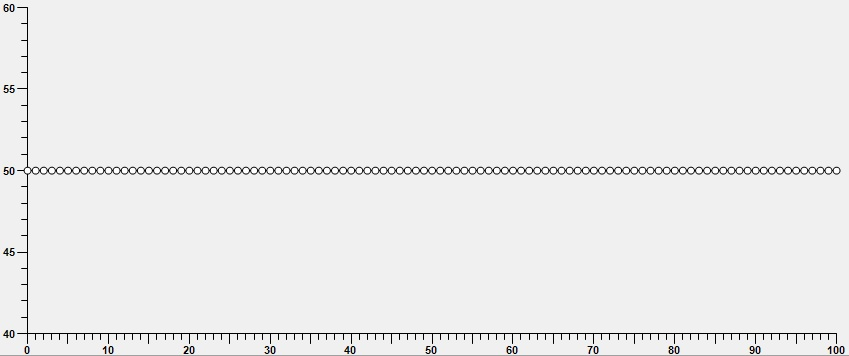Sine wave X: \$IY: SIN(\$I/10)*10Flat multilevel recurring X: \$IY: Mod(\$I/3,5) Function Mod(A,B) returns the modulus ('integer leftover') of A divided by B in Euclidean fashion This is highly useful function in generating repeating waveforms. Simplified, the MOD(\$I/X, Y) generates pattern of X repeats per level and Y levels.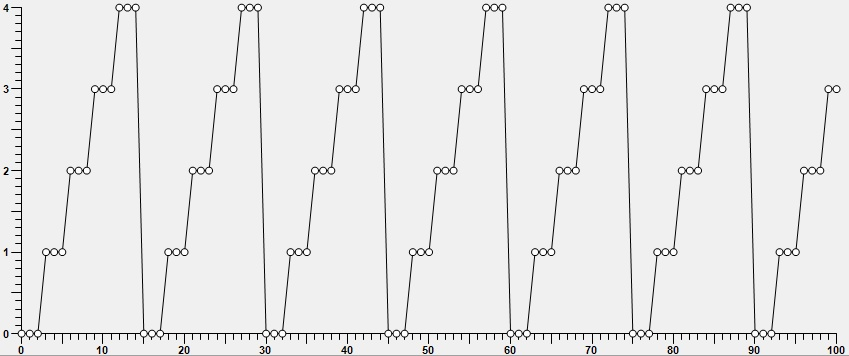Using IF for safety X: \$IY: IF(\$I<80, Mod(\$I/10,3)+(\$I/10), 0) Function IF(A,B,C). If A is 0, return C, else return B.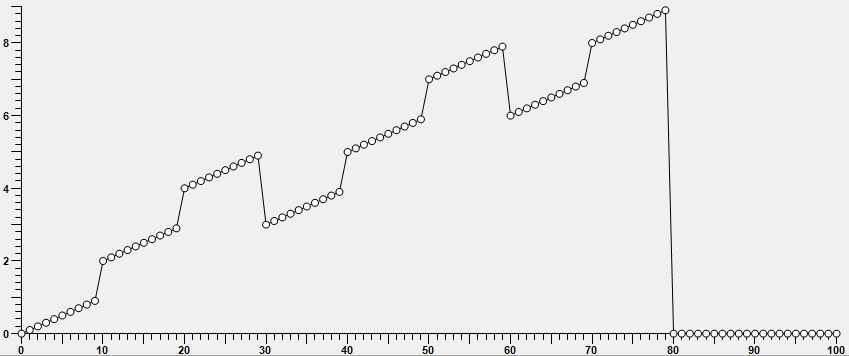Progressive power step X: \$IY: POW(MOD(\$I/5,10), 2.5) Mod expression to power of 2.5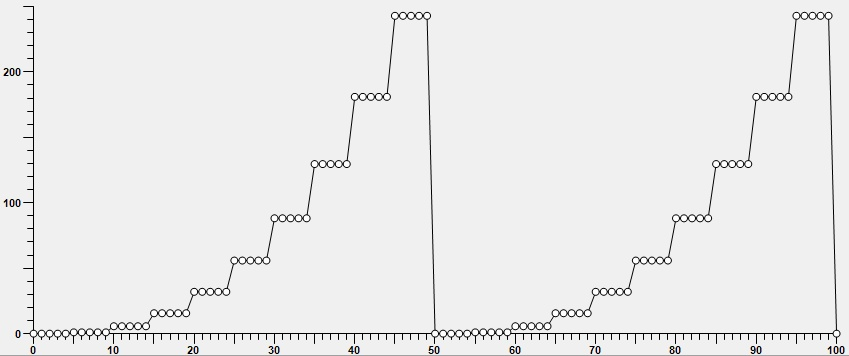Saw X: \$IY: IF(MOD(\$I/40,2)=0, MOD(\$I,40), ABS(40-MOD(\$I,40))) Index 0-200Bias or potential step X: \$I Y: (MOD(\$I/60,5)+1)*(MOD((\$I+20)/20,3)-1) Instead of \$I, the elapsed minutes can be used: \$N1.TM